#### You may also like### Always Two

Find all the triples of numbers a, b, c such that each one of them plus the product of the other two is always 2.### Not Continued Fractions

Which rational numbers cannot be written in the form x + 1/(y + 1/z) where x, y and z are integers?### Coffee

To make 11 kilograms of this blend of coffee costs £15 per kilogram. The blend uses more Brazilian, Kenyan and Mocha coffee... How many kilograms of each type of coffee are used?

# Square to a Rectangle

##### Age 14 to 16 Short Challenge Level:

Answer: 10 tiles by 17 tiles

Finding an expression for the change in the number of tiles
Original patio: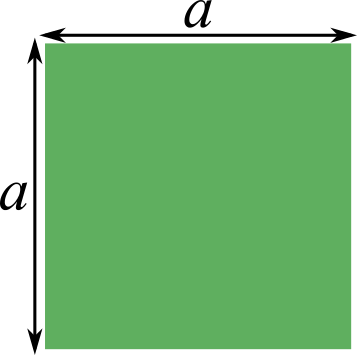Shorten one side by 3 tiles: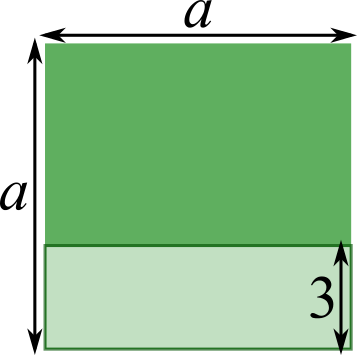Removed 3$a$ tiles

Other side 4 tiles longer: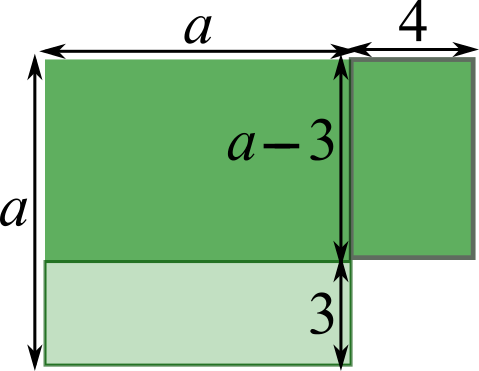Added 4$(a-$3$)$ tiles.

Needed 1 extra tile to make this change, so 4$(a-$3$)$ = 3$a$ + 1, so 4$a-$12 = 3$a$ + 1, so $a-$12 = 1, so $a$ = 13.

13 tiles by 13 tiles square $\Rightarrow$ 10 tiles by 17 tiles rectangle.

Using quadratic expressions for the total area before and after
Before:                                   After: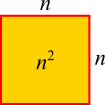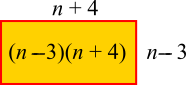The new arrangement uses one extra tile, so \begin{align}n^2+1&=(n-3)(n+4)\\ n^2+1&=n^2+4n-3n-12\\ n^2+1&=n^2+n-12\\ 1&=n-12\\ 13&=n\end{align}
So Kevin had a $13$ tiles by $13$ tiles square, which means that he made a $10$ tiles by $17$ tiles rectangle.

Trying out numbers
The patio used to be a square, so we can test some square numbers to see whether they work.

If the patio started as a 10 tiles by 10 tiles square, then Kevin would have had 100 tiles.
Making one side 3 tiles shorter and the other side 4 tiles longer would have given him a 7 by 14 rectangle.
7$\times$14 = 98, so Kevin would have had 2 spare tiles if he had started off with a 10 by 10 square. But Kevin needed 1 extra tile, so that can't be right.

If the patio started as a 9 tiles by 9 tiles square, then Kevin would have had 81 tiles.
Making one side 3 tiles shorter and the other side 4 tiles longer would have given him a 6 by 13 rectangle.
6$\times$13 = 78, so Kevin would have had 3 spare tiles if he had started off with a 9 by 9 square. That is worse than the 10 by 10 square, because the 10 by 10 square would only have given Kevin 2 spare tiles. So Kevin's patio must have started as a square that was larger than 10 tiles by 10 tiles.

If the patio started as a 12 tiles by 12 tiles square, then Kevin would have had 144 tiles.
Making one side 3 tiles shorter and the other side 4 tiles longer would have given him a 9 by 16 rectangle.
9$\times$16 = 144, so Kevin would have had exactly the right number of tiles if he started off with a 12 by 12 square and wouldn't have needed to buy 1 extra tile.

If the patio started as a 13 tiles by 13 tiles square, then Kevin would have had 169 tiles.
Making one side 3 tiles shorter and the other side 4 tiles longer would give him a 10 by 17 rectangle.
10$\times$17 = 170, so Kevin would need 1 extra tile to make the rectangle - which is right!
So he must have made a 10 tiles by 17 tiles rectangle.

You can find more short problems, arranged by curriculum topic, in our short problems collection.